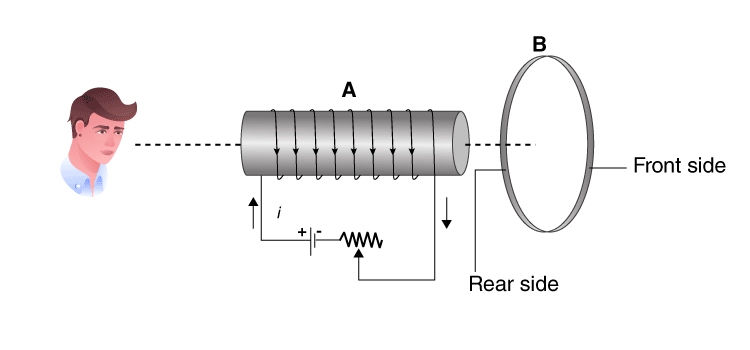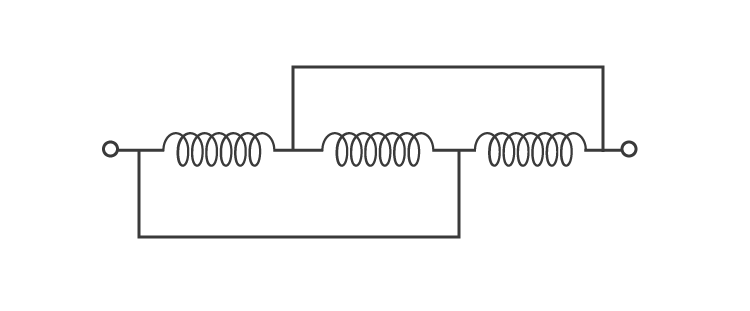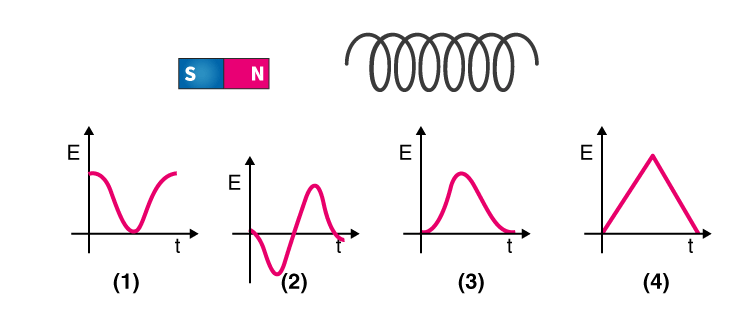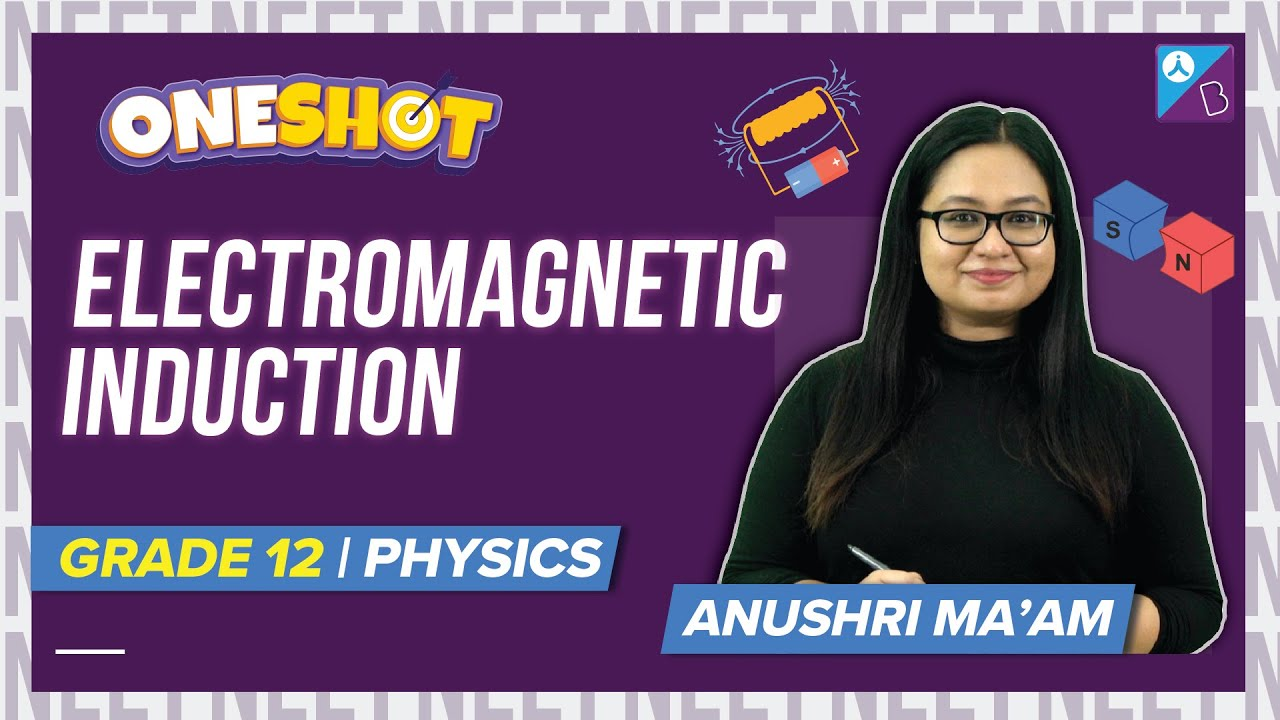Win up to 100% scholarship on Aakash BYJU'S JEE/NEET courses with ABNAT Win up to 100% scholarship on Aakash BYJU'S JEE/NEET courses with ABNAT

# Electromagnetic Induction and Alternating Currents MCQs for NEET

Electromagnetic induction is defined as the production of an electromotive force across an electric conductor in the changing magnetic field. The discovery of induction was done by Michael Faraday in the year 1831. Electromagnetic induction finds many applications such as in electrical components which includes transformers, inductors, and other devices such as electric motors and generators.

Alternating current is defined as an electric current which reverses in direction periodically. In most of the electric power circuits, the waveform of alternating current is the sine wave.

1. How to increase the energy stored in an inductor by four times?
(a) By doubling the current
(b) This is not possible
(c) By doubling the inductance

$$\begin{array}{l}(d) \ By \ making \ current \ \sqrt{2} \ times\end{array}$$

Answer: (a) By doubling the current

2. Consider an inductor whose linear dimensions are tripled and the total number of turns per unit length is kept constant, what happens to the self-inductance?
(a) 9 times
(b) 3 times
(c) 27 times

$$\begin{array}{l}(d) \frac{1}{3} times\end{array}$$

3. Lenz law is based on which of the following conservation>
(a) Charge
(b) Mass
(c) Momentum
(d) Energy

4. What will be the acceleration of the falling bar magnet which passes through the ring such that the ring is held horizontally and the bar magnet is dropped along the axis of the ring?

(a) It depends on the diameter of the ring and the length of the magnet
(b) It is equal due to gravity
(c) It is less than due to gravity
(d) It is more than due to gravity

Answer: (c) It is less than due to gravity

5. An observer is seeing the setup of an aluminium ring B facing an electromagnet A. The current I through A can be altered if(a) B is independent of an increase or decrease of I
(b) If I increases, A will attract B
(c) If I increase, A will repel B
(d) If I decrease, A will repel B

Answer: (c) If I increase, A will repel B

6. What is the equivalent inductance of the following circuit if the pure inductance is 3.0H(a) 9H
(b) 3H
(c) 1H
(d) 2H

7. What is the need for laminating the core of a transformer?
(a) To reduce the resistance in the winding
(b) To reduce the eddy currents
(c) To reduce the hysteresis
(d) None of the above

Answer: (b) To reduce the eddy currents

8. Which of the following represent the variation of induced EMF with respect to time t if a short bar magnet is moved along its axis and has a constant velocity.9. A wire loop is rotated in a magnetic field such that the frequency of change of direction of the induced emf is
(a) Six times per revolution
(b) Four times per revolution
(c) Twice per revolution
(d) Once per revolution

10. Which of the following is the equivalent quantity of mass in electricity?
(a) Current
(b) Charge
(c) Potential
(d) Inductance

11. What is the unit of inductance?
(a) Volt/ampere
(b) Joule/ampere
(c) Volt-ampere/sec
(d) Volt-sec/ampere

12. What is the angle made by the plane of eddy currents with the plane of magnetic lines of force?
(a) 0°
(b) 40°
(c) 90°
(d) 180°